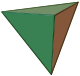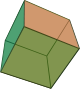Consider all solids with faces that are regular polygons, and where the vertices have the same number of faces. There are five, and they are called the Platonic Solids, after Plato.

Proof that there are exactly five is an interesting exercise. This is related to graph theory and the Euler characteristic.TetrahedronHexahedron (or cube, to you and me)OctahedronDodecahedronIcosahedron